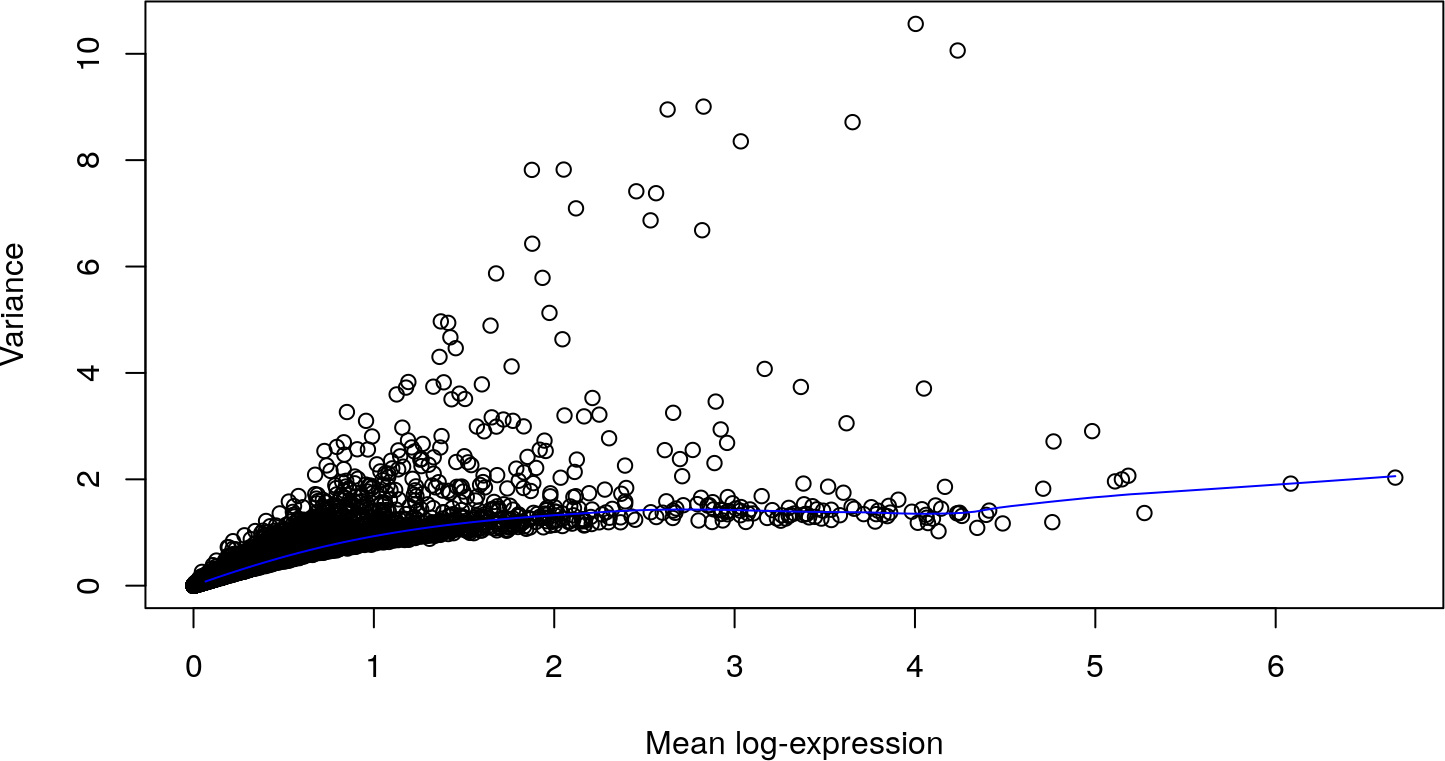# 1 Introduction

Single-cell RNA sequencing (scRNA-seq) is a widely used technique for profiling gene expression in individual cells. This allows molecular biology to be studied at a resolution that cannot be matched by bulk sequencing of cell populations. The scran package implements methods to perform low-level processing of scRNA-seq data, including cell cycle phase assignment, scaling normalization, variance modelling and testing for corrrelated genes. This vignette provides brief descriptions of these methods and some toy examples to demonstrate their use.

Note: A more comprehensive description of the use of scran (along with other packages) in a scRNA-seq analysis workflow is available at https://osca.bioconductor.org.

# 2 Setting up the data

We start off with a count matrix where each row is a gene and each column is a cell. These can be obtained by mapping read sequences to a reference genome, and then counting the number of reads mapped to the exons of each gene. (See, for example, the Rsubread package to do both of these tasks.) Alternatively, pseudo-alignment methods can be used to quantify the abundance of each transcript in each cell. For simplicity, we will pull out an existing dataset from the scRNAseq package.

``````library(scRNAseq)
sce <- GrunPancreasData()
sce``````
``````## class: SingleCellExperiment
## dim: 20064 1728
## assays(1): counts
## rownames(20064): A1BG-AS1__chr19 A1BG__chr19 ... ZZEF1__chr17
##   ZZZ3__chr1
## rowData names(2): symbol chr
## colnames(1728): D2ex_1 D2ex_2 ... D17TGFB_95 D17TGFB_96
## colData names(2): donor sample
## reducedDimNames(0):
## spikeNames(0):
## altExpNames(1): ERCC``````

This particular dataset is taken from a study of the human pancreas with the CEL-seq protocol (Grun et al. 2016). It is provided as a `SingleCellExperiment` object (from the SingleCellExperiment package), which contains the raw data and various annotations. We perform some cursory quality control to remove cells with low total counts or high spike-in percentages:

``````library(scater)
qcstats <- perCellQCMetrics(sce)
qcfilter <- quickPerCellQC(qcstats, percent_subsets="altexps_ERCC_percent")
``````##    Mode   FALSE    TRUE
## logical    1291     437``````

# 3 Normalizing cell-specific biases

## 3.1 Based on the gene counts

Cell-specific biases are normalized using the `computeSumFactors` method, which implements the deconvolution strategy for scaling normalization (A. T. Lun, Bach, and Marioni 2016). This computes size factors that are used to scale the counts in each cell. The assumption is that most genes are not differentially expressed (DE) between cells, such that any differences in expression across the majority of genes represents some technical bias that should be removed.

``````library(scran)
clusters <- quickCluster(sce)
sce <- computeSumFactors(sce, clusters=clusters)
summary(sizeFactors(sce))``````
``````##     Min.  1st Qu.   Median     Mean  3rd Qu.     Max.
## 0.006722 0.442629 0.801312 1.000000 1.295809 9.227575``````

For larger data sets, clustering should be performed with the `quickCluster` function before normalization. Briefly, cells are grouped into clusters of similar expression; normalization is applied within each cluster to compute size factors for each cell; and the factors are rescaled by normalization between clusters. This reduces the risk of violating the above assumption when many genes are DE between clusters in a heterogeneous population.

Note that `computeSumFactors` will automatically remove low-abundance genes, which provides some protection against zero or negative size factor estimates. We also assume that quality control on the cells has already been performed, as low-quality cells with few expressed genes can often have negative size factor estimates.

## 3.2 Based on the spike-in counts

An alternative approach is to normalize based on the spike-in counts (Lun et al. 2017). The idea is that the same quantity of spike-in RNA was added to each cell prior to library preparation. Size factors are computed to scale the counts such that the total coverage of the spike-in transcripts is equal across cells. The main practical difference is that spike-in normalization preserves differences in total RNA content between cells, whereas `computeSumFactors` and other non-DE methods do not.

``````sce2 <- computeSpikeFactors(sce, "ERCC")
summary(sizeFactors(sce2))``````
``````##    Min. 1st Qu.  Median    Mean 3rd Qu.    Max.
## 0.01041 0.57760 0.88667 1.00000 1.27679 7.43466``````

## 3.3 Computing normalized expression values

Normalized expression values are calculated using the `logNormCounts()` method from scater (McCarthy et al. 2017). This will use the deconvolution size factors for the endogenous genes, and the spike-in-based size factors for the spike-in transcripts. Each expression value can be interpreted as a log-transformed “normalized count”, and can be used in downstream applications like clustering or dimensionality reduction.

``sce <- logNormCounts(sce)``

# 4 Variance modelling

We identify genes that drive biological heterogeneity in the data set by modelling the per-gene variance. The aim is use a subset of highly variable genes in downstream analyses like clustering, to improve resolution by removing genes driven by technical noise. We decompose the total variance of each gene into its biological and technical components by fitting a trend to the endogenous variances (A. T. Lun, McCarthy, and Marioni 2016). The fitted value of the trend is used as an estimate of the technical component, and we subtract the fitted value from the total variance to obtain the biological component for each gene.

``````dec <- modelGeneVar(sce)
plot(dec\$mean, dec\$total, xlab="Mean log-expression", ylab="Variance")``````dec2 <- modelGeneVarWithSpikes(sce, 'ERCC')